# Microsoft SQL Interview Questions - Freemium vs Premium

In this blog, we will walk through a SQL interview question that was asked by Microsoft. Preparing such SQL interview questions will surely help you to ace your data science interview.

This Microsoft SQL interview question has multiple steps including applying a LEFT JOIN to 3 database tables, using a CASE WHEN to categorize data, and using the SQL sum() function to count downloads. We then use a SUBQUERY and apply a WHERE (and we'll also talk about how to use a HAVING SQL clause) to filter records down to what you’re looking for. We finish off the question by using an ORDER BY and outputting only the 3 columns of interest. This question covers concepts that are commonly found in data science interviews at Microsoft, Facebook and Google.

## Microsoft SQL Interview Question

Now, we will take a look at the SQL interview question that was asked in a data science interview at Microsoft.

Premium vs Freemium

Find the total number of downloads for paying and non-paying users by date. Include only records where non-paying customers have more downloads than paying customers. The output should be sorted by earliest date first and contain 3 columns date, non-paying downloads, paying downloads.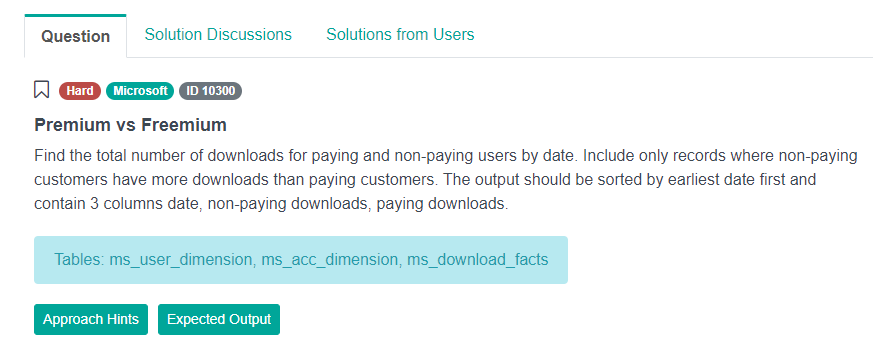Link to the question: https://platform.stratascratch.com/coding-question?id=10300&python=

### Exploring the Data

The first thing we should do is check out the data. There are three tables. Let's first go to the download_facts table.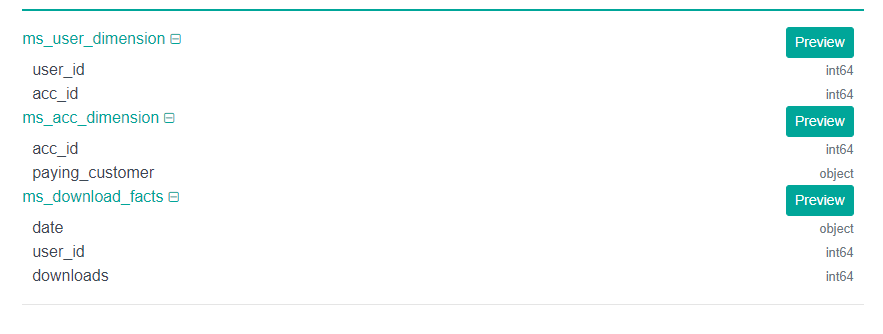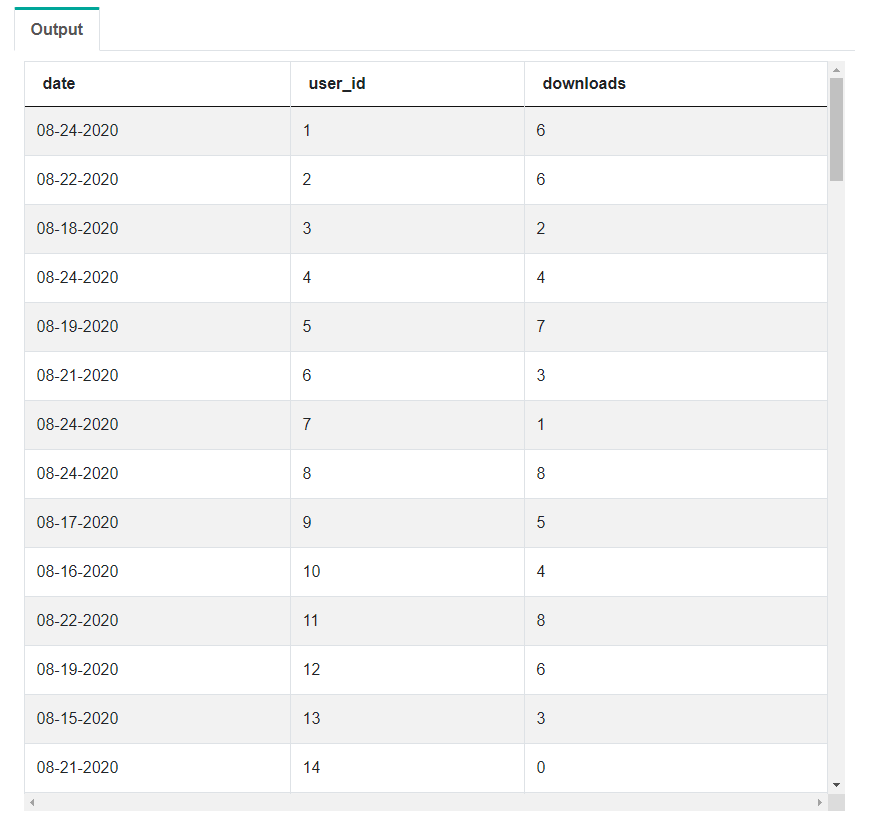When we click the preview button, we can see the number of downloads, the user_id and the date. So, presumably the number of downloads that the user performed on that specific date.

Then, let's go to the account_dim table.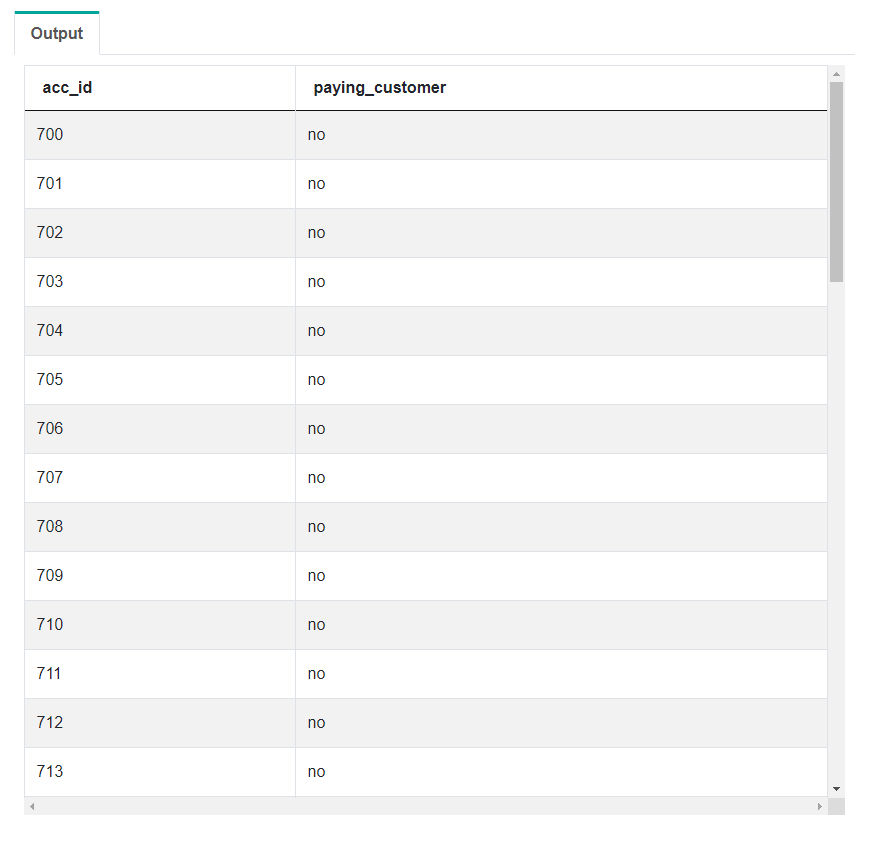Now, we see account_id and paying_customer. Paying_customer is the column we'll use to classify whether or not the user is a paying user or a non-paying user according to the question.

The last table is the user_dim table.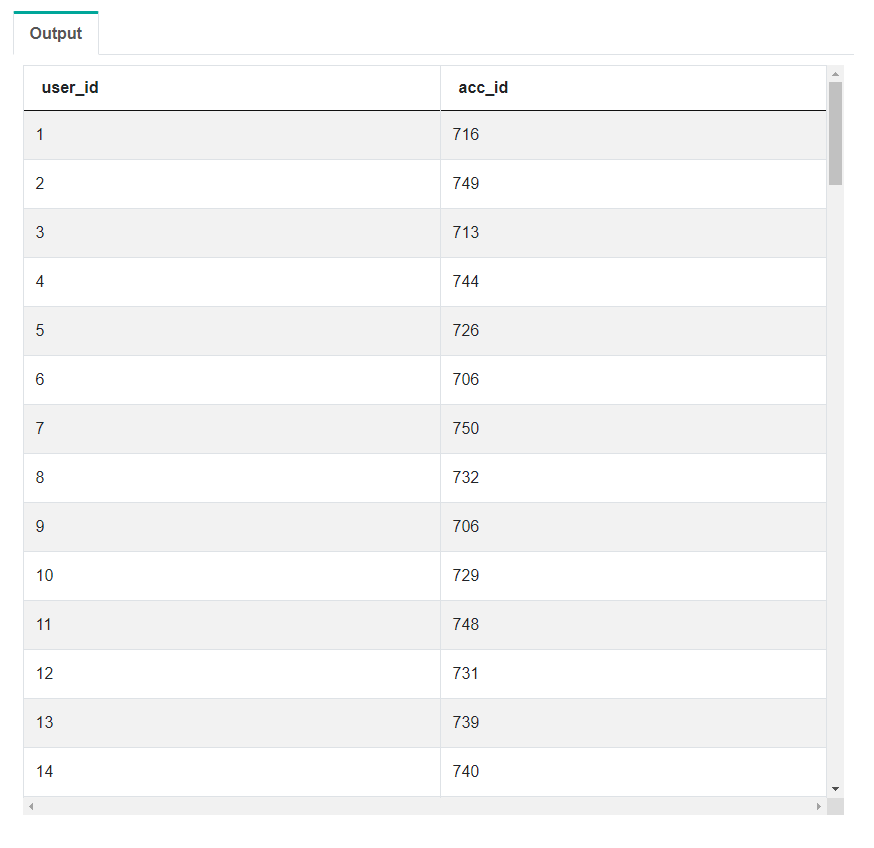In this table, we see the user_id and the associated account_id. We can consider this as the master table and we can join the account_dim and the download_facts table to this table.

The next thing in the process of solving this Microsoft SQL interview question is we'll talk about the approach and the assumptions we have with the data.

### Developing the Approach

What this Microsoft SQL interview question is asking us to do is sum up all of the downloads by the user and then categorize that by a paying customer or a non-paying customer, and then group by date. In order to do that, we have to traverse through the three tables we have to get all the information that we need.

### JOIN Table Logic

The first thing we want to do to solve this Microsoft SQL interview problem is to join the three tables together.

``-- join three tables together using INNER JOIN/LEFT JOIN``

We need to figure out if we should use an INNER JOIN or a LEFT JOIN to join all these tables together. To figure that out, we need to take a look at a few of the tables first.

#### 1. user_dimension

Let's take a look at this user_dim table where we have this user_id and account_id. And this is just a mapping that allows us to link the other two tables together. We're going to assume that this table is a master list of all user IDs and their associated accounts. So, we have all the user IDs there.

#### 2. download_facts

If we take a look at the download_facts table. We have a user_id and downloads. Presumably, this is all the users that have performed a download. But it doesn't include users that have not performed a download. But the user_dim table above has a list of all of the users, both that have performed a download and not perform a download.

If we LEFT JOIN the tables together, you might get two rows that look like this.

``````-- join three tables together using INNER JOIN/LEFT JOIN

-- user_id | acc_id |    date    | user_id | downloads
--    123      AAA    01-01-2020     123        6
--    321      BBB      NULL       NULL      NULL``````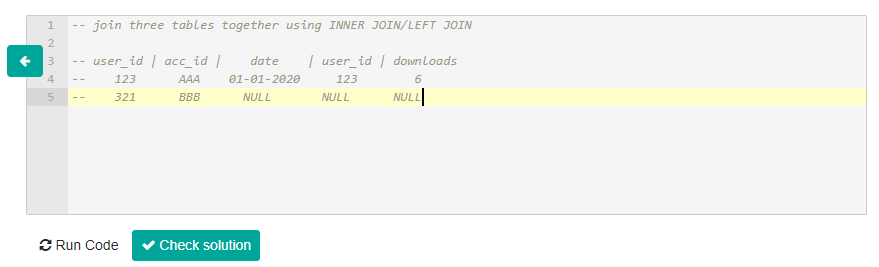What we see is if a user performs a download you get a full set of values across all the columns. You get user 123 that performed six downloads. Then in the second row, we have a user_id 321 that did not perform any downloads. And you get a bunch of nulls because there isn't a match there.

Now, the question is are those nulls going to impact and affect the output? And the answer is NO because we want to sum up all the downloads using a sum function. And it will just skip these nulls and only sum up values that it sees in the 'downloads' column.

So we can use a LEFT JOIN if we want. In addition to that, we can also use an INNER JOIN. If we use the INNER JOIN on these two tables, what happens is the last row will be removed.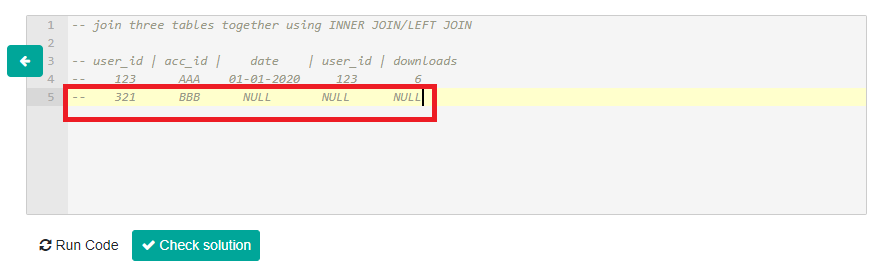We can use an INNER JOIN as well. In this case, we're just going to use a LEFT JOIN.

### CASE WHEN Logic

Once we join the three tables together, the next step is to categorize whether a user is a paying customer or not. Now, we have to leverage the account_dim table and the specific column 'paying_customer'. A paying customer is a paying customer if the value is 'yes'. They're not a paying customer if the value is 'no'. So in this case, we have to use a CASE WHEN to classify these users.

``-- categorize users using a CASE WHEN on paying_customer``

### sum() on CASE WHEN Logic

Once we are able to categorize customers, we have to sum up their downloads. So, we are going to use a sum function and apply that to the CASE WHEN clause.

``-- sum() on CASE WHEN``

### SUBQUERY and WHERE Logic

Now, we have to filter out records where paying customers have greater downloads than non-paying customers. So, we want to keep records where the non-paying customer downloads are greater than the paying customer downloads. In order to do this, we need to utilize a subquery and then perform that logic outside of that subquery.

``````-- SUBQUERY and use WHERE clause to keep records where
non-paying customer``````

### ORDER BY Logic

Lastly, we have to group by date and then order the records by earliest date to the latest date, and then output the three columns that the question is asked.

``````-- ORDER BY earliest date
-- Output date, non-paying, paying``````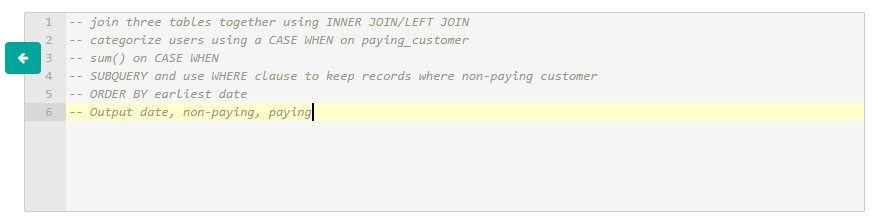These were our steps to solve this Microsoft SQL interview question. And the next thing we want to do is just start coding from the first step down to the last step.

### Coding - LEFT JOINs

Now, Let's join the three tables together using a LEFT JOIN.

``````SELECT
*
FROM ms_user_dimension mud
LEFT JOIN ms_acc_dimension mad ON mad.acc_id = mud.acc_id
LEFT JOIN ms_download_facts mdf ON mdf.user_id = mdf.user_id``````

Here we LEFT JOIN the account_dim and the downloads_facts table to the user_dim table. The user_dim table is what we're considering the master table that has all of the user IDs and all of the account IDs. And if we run this query, we have all of the columns in all three tables displayed out here.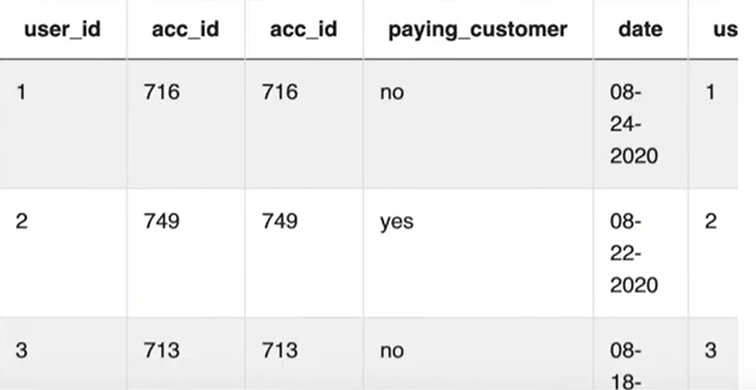### Coding - CASE WHEN

The next thing we have to do is use a CASE WHEN to categorize whether a user is a paid customer or not a paying customer using the column called 'paying_customer'.

``````SELECT
CASE WHEN paying_customer = 'yes' THEN downloads END as paying,
CASE WHEN paying_customer = 'no' THEN downloads END as non_paying
FROM ms_user_dimension mud
LEFT JOIN ms_acc_dimension mad ON mad.acc_id = mud.acc_id
LEFT JOIN ms_download_facts mdf ON mdf.user_id = mdf.user_id``````

What this CASE WHEN does is it will take the paying_customer column and extracts the 'yes' and if it finds a 'yes', it will then output the number of downloads that user performed. If the paying customer value is 'no', we also extract the number of downloads from that user and categorize that as a non-paying customer.

### Coding - sum()

Now, we want to sum up the total amount of downloads by paying customer and non-paying customer. Let's just implement the sum function around this case one.

``````SELECT
sum(CASE WHEN paying_customer = 'yes' THEN downloads END)
as paying,
sum(CASE WHEN paying_customer = 'no' THEN downloads END)
as non_paying
FROM ms_user_dimension mud
LEFT JOIN ms_acc_dimension mad ON mad.acc_id = mud.acc_id
LEFT JOIN ms_download_facts mdf ON mdf.user_id = mdf.user_id``````

When we run this query, we get the total amount of downloads by paying customer versus the non-paying customer.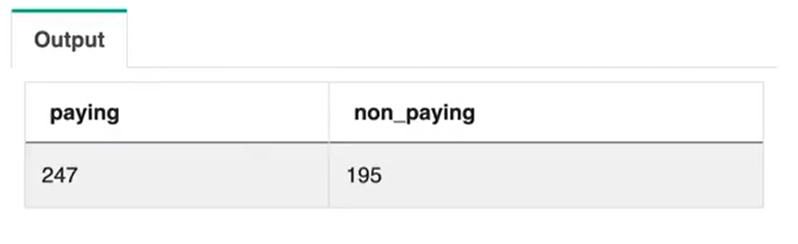Now, we want to see this breakdown by date. So, let's add the date to the SELECT clause and then add a group by.

``````SELECT
date,
sum(CASE WHEN paying_customer = 'yes' THEN downloads END)
as paying,
sum(CASE WHEN paying_customer = 'no' THEN downloads END)
as non_paying
FROM ms_user_dimension mud
LEFT JOIN ms_acc_dimension mad ON mad.acc_id = mud.acc_id
LEFT JOIN ms_download_facts mdf ON mdf.user_id = mdf.user_id
GROUP BY date``````

Let's run this query now.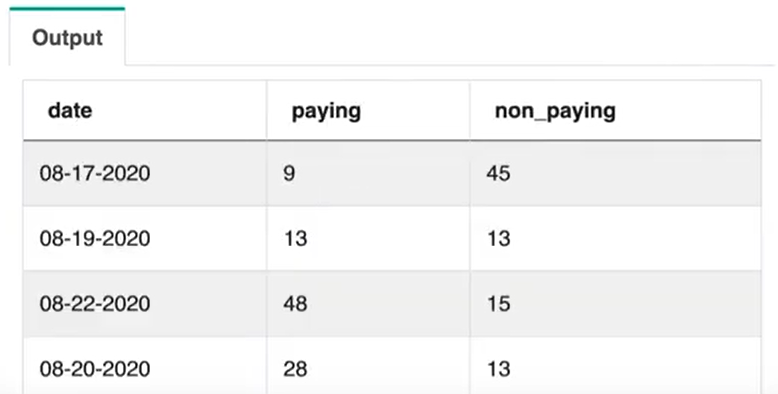So, we got downloads by paying customer and non-paying customer by date.

### Coding - SUBQUERY & WHERE

The next step is to keep records where the non-paying downloads are greater than the paying downloads. To do that, our query needs to be a subquery or it needs to be put into a temp table. And then we can apply that logic on top of that temp table or subquery. After using a subquery, we'll apply that logic in the where clause.

``````SELECT
*
FROM (
SELECT
date,
sum(CASE WHEN paying_customer = 'yes' THEN downloads END)
as paying,
sum(CASE WHEN paying_customer = 'no' THEN downloads END)
as non_paying
FROM ms_user_dimension mud
LEFT JOIN ms_acc_dimension mad ON mad.acc_id = mud.acc_id
LEFT JOIN ms_download_facts mdf ON mdf.user_id = mdf.user_id
GROUP BY date) t
WHERE paying < non_paying``````

If we run this query, we get the rows where the number of downloads for a non-paying user is greater than the number of downloads for a paying user.

### Coding - ORDER BY

Now, we want to ORDER BY earliest date. As you can see the output is mixed up right now, so we could apply this in the subquery, where we ORDER BY date ascending, because we want the earliest date first. And then on top, we just output the date, the non-paying download number, and then the paying download number.

``````SELECT
date,
non_paying,
paying
FROM (
SELECT
date,
sum(CASE WHEN paying_customer = 'yes' THEN downloads END)
as paying,
sum(CASE WHEN paying_customer = 'no' THEN downloads END)
as non_paying
FROM ms_user_dimension mud
LEFT JOIN ms_acc_dimension mad ON mad.acc_id = mud.acc_id
LEFT JOIN ms_download_facts mdf ON mdf.user_id = mdf.user_id
GROUP BY date
ORDER BY date ASC) t
WHERE paying < non_paying``````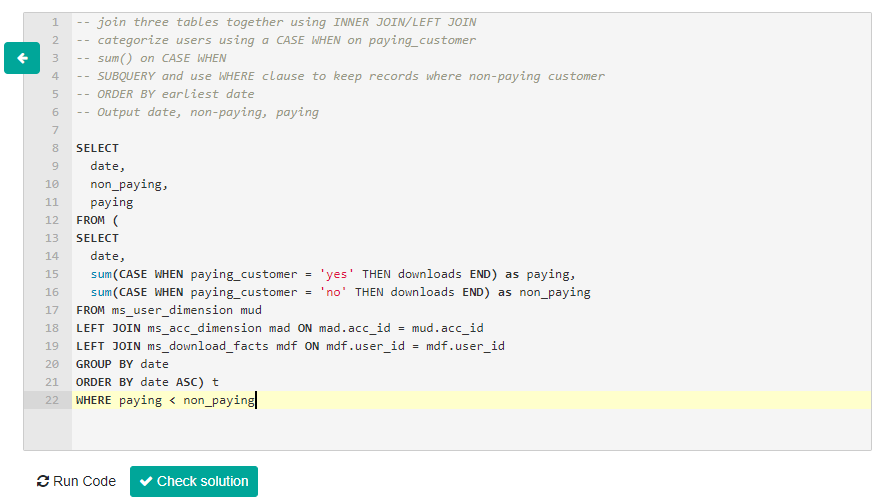Let's run this code now.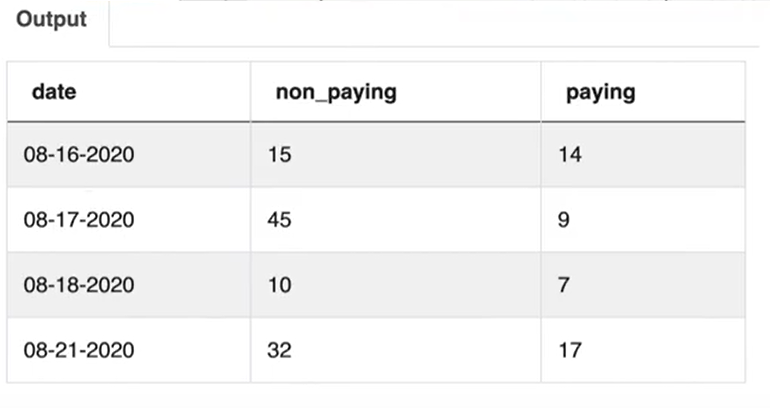This is the answer to this Microsoft SQL interview question. We can also check this solution on StrataScratch platform.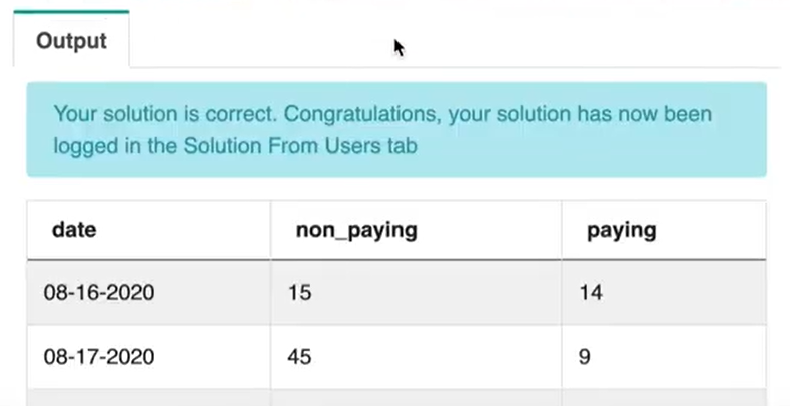#### Coding - Using HAVING as Alternative

I do want to show you one other way of solving this Microsoft SQL interview question instead of using a WHERE clause. I want to show you how to use a HAVING clause that will get you to the same output. Instead of having a WHERE clause, we can utilize a HAVING clause. And then we can take the difference between non-paying and paying and just ensure that is greater than zero.

``````SELECT
date,
non_paying,
paying
FROM (
SELECT
date,
sum(CASE WHEN paying_customer = 'yes' THEN downloads END)
as paying,
sum(CASE WHEN paying_customer = 'no' THEN downloads END)
as non_paying
FROM ms_user_dimension mud
LEFT JOIN ms_acc_dimension mad ON mad.acc_id = mud.acc_id
LEFT JOIN ms_download_facts mdf ON mdf.user_id = mdf.user_id
GROUP BY date
ORDER BY date ASC) t
HAVING (non_paying - paying) > 0``````

This logic means that the number of downloads from a non-paying customer has to be greater than the number of downloads by a paying customer. And if that is true, the difference will be greater than zero.

Another clause to add is a GROUP BY clause. In addition to this HAVING clause, we will add the GROUP BY clause above the HAVING clause. And use the column 'date', 'non-paying' and 'paying'.

``````SELECT
date,
non_paying,
paying
FROM (
SELECT
date,
sum(CASE WHEN paying_customer = 'yes' THEN downloads END)
as paying,
sum(CASE WHEN paying_customer = 'no' THEN downloads END)
as non_paying
FROM ms_user_dimension mud
LEFT JOIN ms_acc_dimension mad ON mad.acc_id = mud.acc_id
LEFT JOIN ms_download_facts mdf ON mdf.user_id = mdf.user_id
GROUP BY date
ORDER BY date ASC) t
GROUP BY date, non_paying, paying
HAVING (non_paying - paying) > 0``````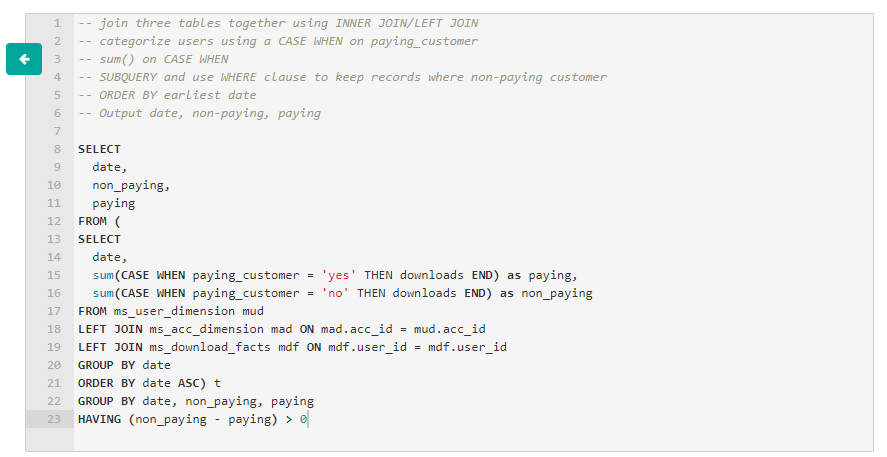If we run this query, we get the same output as when we used a WHERE clause.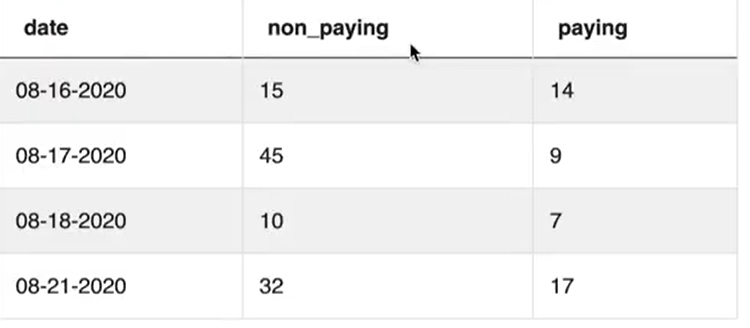That's just another way to solve this Microsoft SQL interview question. If you don't want to use a WHERE, you can use a HAVING clause. It makes the code a little bit longer because you have to add a GROUP BY but in the end, you will be getting the same output.

Want more interview questions and solutions from Microsoft? Check out more blogs:

Strata Scratch, LLC © 2021
team@stratascratch.com